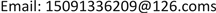HJCEHans Journal of Civil Engineering2326-3458Scientific Research Publishing10.12677/HJCE.2018.75080HJCE-26671HJCE20180500000_67577405.pdf工程技术 基于精细化控制的供热系统节能分析 Energy Saving Analysis of Heat Supply System Based on Elaborate Control 玉才21314*长安大学，陕西 西安西安新鸿业投资发展有限公司，陕西 西安中铁西安勘察设计研究院有限责任公司，陕西 西安null020820180705678685© Copyright 2014 by authors and Scientific Research Publishing Inc. 2014This work is licensed under the Creative Commons Attribution International License (CC BY). http://creativecommons.org/licenses/by/4.0/

1西安新鸿业投资发展有限公司，陕西 西安

2中铁西安勘察设计研究院有限责任公司，陕西 西安

3长安大学，陕西 西安Copyright © 2018 by authors and Hans Publishers Inc.1. 引言

2. 理论耗热量

2.1. 住宅建筑

q = 20 − 2.6 18 − ( − 3.4 ) × 40 = 32.52 W/m2

Q = 32.52 × 120 × 24 × 3600 / 10 9 = 0.3372 GJ/m2

2.2. 办公建筑

q = [ 20 − 2.6 18 − ( − 3.4 ) × 15 24 + 5 − 2.6 18 − ( − 3.4 ) × 9 24 ] × 60 = 33.01 W/m2

Q = 33.01 × 120 × 24 × 3600 / 10 9 = 0.3422 GJ/m2

Q = 60 × 120 × 24 × 3600 / 10 9 = 0.6221 GJ/m2

2.3. 宾馆建筑

q = 22 − 2.6 18 − ( − 3.4 ) × 45 = 40.79 W/m2

Q = 40.79 × 120 × 24 × 3600 / 10 9 = 0.4229 GJ/m2

2.4. 工业建筑

q = [ 20 − 2.6 18 − ( − 3.4 ) × 15 24 + 5 − 2.6 18 − ( − 3.4 ) × 9 24 ] × 50 = 27.51 W/m2

Q = 27.51 × 120 × 24 × 3600 / 10 9 = 0.2852 GJ/m2

3. 集中供热系统精细化控制3.1. 分布式变频系统设计3.1.1. 分布式变频系统原理

3.1.2. 分布式变频系统设计

1) 管网系统进行水力计算，确定系统所需的流量和压力分布。

2) 按照供热的经济性，合理选择压差控制点位置，一般控制在热源厂内部。

3) 选择主循环泵，流量满足全网流量需求，扬程克服压差控制点之前的系统阻力。

4) 选择用户一次水泵，满足该分支用户的阻力和流量。用户一次水泵应满足用户近、远期负荷发展要求，并能适应外网压力变化。

3.2. 集中供热系统精细化控制3.2.1. 负荷预测

3.2.2. 检测参数

3.2.3. 控制对象

3.2.4. 控制方法

t p j = t g + t h 2 = q h q h 0 ( t p j 0 − t n 0 ) + t n (1)

( τ 1 − t g ) − ( τ 2 − t h ) lg τ 1 − t g τ 2 − t h = ( τ 10 − t g 0 ) − ( τ 20 − t h 0 ) lg τ 10 − t g 0 τ 20 − t h 0 q h q h 0 (2)

4. 运行效果分析4.1. 设计条件

2016~2017年采暖季共有4家用户投入运行，分别为第一学校、第六小学、公安局、消防站。各换热站设计条件见表1 (其中，第一学校换热站夜间仅维持5℃的值班温度)。

4.2. 运行效果

2016~2017年采暖季的实际运行效果表明，基于精细化控制的供热系统具有十分可观的节能潜力。对于夜间仅维持值班温度的热用户(第一学校)，节能潜力尤为可观(表2)。表中所有用户的实际耗热量均小于理论耗热量，平均实际耗热量指标为0.1569 GJ/m2，平均实际热负荷仅15.13 W/m2

Design conditions of heating users in 2016-201

1第一学校3.48191580/601.19
2第六小学5.25251575/503.27
3公安局1.5770585/600.98
4消防站0.8042050/400.50

Operating results of heating users in 2016-201

1第一学校1.190.290.08468.16
2第六小学3.271.060.201519.43
3公安局0.980.230.147214.20
4消防站0.500.160.197519.05

5. 结论与建议